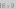# Project 2 - Transformations

In this project we will implement transformations using JavaScript.

You are given an HTML file that implements a very simple UAV simulation. The video below shows what the finished project should look like.The missing part of this application (the part you will implement) consists of two JavaScript function. The first one, `GetTransform`, returns a 3x3 transformation matrix defined by the given transformation arguments. Correctly implementing this function is sufficient for applying the correct transformation to the UAV body. Here what this function looks like:

```function GetTransform( positionX, positionY, rotation, scale )
```

This function takes 4 input parameters: `positionX` and `positionY` define the translation component and the other two parameters define the `rotation` (in degrees) and the `scale` components. The returned transformation should first apply scale, then rotation, and finally translation. The transformation is matrix is returned as a 1D array of values in column-major format, such that the array indices correspond to the matrix values as below:

 `array` `array` `array` `array` `array` `array` `array` `array` `array`

The second function you should implement, `ApplyTransform`, takes two 3x3 transformation matrices and returns the resulting transformation as a combined 3x3 transformation matrix, all in the same column-major format as above. Here is what this function looks like:

```function ApplyTransform( trans1, trans2 )
```

The returned transformation should first apply `trans1` and then `trans2`. This second function is needed for applying the local transformations of the four propellers before applying the transformation of the UAV body. This is how the propellers are placed at their correct positions.

You are given the following files to help you with this project:

• project2.html: This file contains the entire implementation, except for the two functions you will implement.
• project2.js: This file contains the placeholder for the two functions. It is included by the project2.html file. Please make sure to put them in the same directory.
• The project2.html file also includes a few image files:

Complete the two functions in the project2.js file, such that the UAV moves along with its shadow. Then, submit the completed project2.js file on canvas. Please do not rename project2.js in your submission.